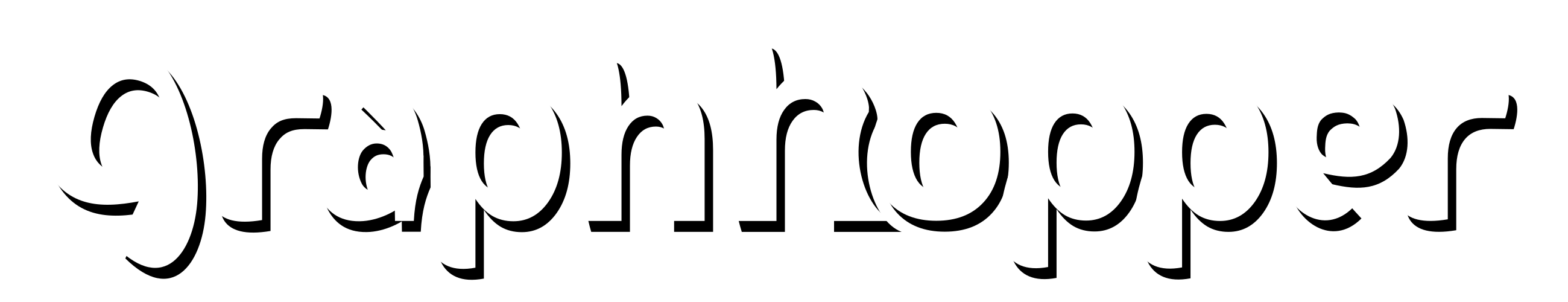# Low level api - DijkstraBidirectionRef

Hiyas,

Just wondering if I am understanding DijkstraBidirectionRef correctly.
Does this calculate both directions between points?
eg. Point A to Point B and Point B to Point A

I can process the path using `path = new DijkstraBidirectionRef(queryGraph, w, EDGE_BASED).calcPath(fromSnap.getClosestNode(), toSnap.getClosestNode());`

But when mapped this only shows the Point A to Point B.
`PointList tmp = path.calcPoints();`

Am I required to also make another path Point B to Point A or do I need to setup an algo using BidirRoutingAlgorithm?

Thanks,

“bidirection” means that the route from A to B is calculated with two Dijkstras instead of one for a faster response (approx. 2x):

• the forward Dijkstra search from A (A -> x_i) and
• the reverse Dijkstra search from B (B <- y_i) and

then both Dijkstra trees will overlap somewhere in the middle and under certain conditions you can stop the search (see the finish method).

To calculate “B to A” you need to call the algorithm again and you get a different forward Dijkstra (B -> x_k) and another Dijkstra (y_k -> A). You could reuse the reverse Dijkstra only if traversing a road segment in both directions leads to the same speed etc (i.e. no oneways, no different estimated speeds etc), i.e. normally you cannot reuse it, not even parts.

1 Like

Ah gotcha. I was confused thinking Bidirection was A <-> B
Makes perfect sense and is really cool!

For peace of mind I think two calls is best.

Thanks for explaining.

1 Like